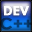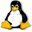Home Latest News Archive Tutorials C++ 2 3 4 Win32 Java OpenGL GLUT OpenGL ES 2 3 4 DirectX VB.NET HTML Photoshop 3dsmax Forums Projects Modelling Links About Us Search Recent Tutorials C / C++ Win32 OpenGL OpenGL ES GLUT## Scope

Tutorials > C++ > Scope

### What is scope?

We have spoken before about a block of code. Anything between { and } is seen as a block of code.
The scope of a variable can be seen as the visibility of the variable. A variable can only be referenced in the block or a sub-block that it was declared in. This is useful as it allows you to encapsulate data and make your programs more modular.

Contents of main.cpp :

```#include <iostream>
#include <stdlib.h>

using namespace std;
```

Any variables not declared in a block ie. declared outside of the main or any other function, is known to be in global scope. This means that the variable can be used anywhere within your source file. The variable x below can therefore be referenced anywhere in our program.

```// Global Scope
//------------------
int x = 5;

int main()
{
int y = 10;
int z = 3;
```

The code below shows how x can now be used, even though it is not declared in the same code block.

```	cout << "global x before block : "
<< x << endl;
```

The code below shows the start of a code block. You will generally see code blocks when dealing with functions, statements(if, switch) and loops(for, while).

```	// Code block
//------------
{
```

Another variable x is now declared. But aren't variable names supposed to be unique? This is true if the variables are within the same scope. If a variable with the same name is declared within another scope, the relevant variable will be referenced depending on what scope you are currently in.

```		int x = 4;
```

The statement x += 5 increases the x variable declared within the code block.

```		cout << "x in block : "
<< x << endl;

x += 5;

cout << "inner x after add : "
<< x << endl;
```

If you happen to want to reference the variable in global scope, you can use the scope resolution operator ::

```		cout << "global x in block : "
<< ::x << endl;
```

The next piece of code shows how variables declared in a parent block can still be used within an inner scope.

```		y += 10;

cout << "y after add : "
<< y << endl;
}
```

When y is printed out, the expected value of 20 is displayed.
You may be surprised that when the variable x is displayed, it has a value of 5. This is because the variable is now referencing the variable in global scope. The variable defined within the code block can now not be referenced as you have left the code block.

```	cout << "y after block : "
<< y << endl;

cout << "x after block : "
<< x << endl;

system("pause");

return 0;
}```

You should now have a basic understanding of what scope is and you should now know when variables can and cannot be referenced.

Please let me know of any comments you may have : Contact Me

 Source Files :< Tutorial 18 - Ternary Operator Tutorial 20 - Functions >